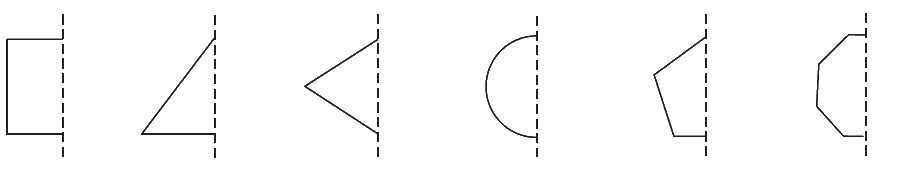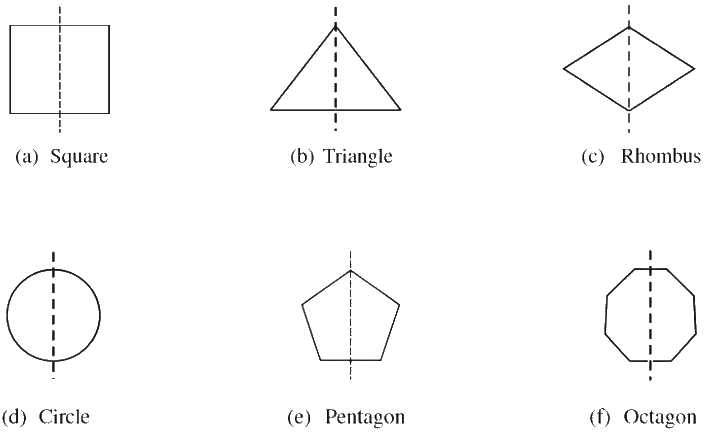# Ex.14.1 Q3 Symmetry - NCERT Maths Class 7

Go back to  'Ex.14.1'

## Question

In the following figures, the mirror line (i.e., the line of symmetry) is given as a dotted line. Complete each figure performing reflection in the $$Q$$ dotted (mirror) line. (You might perhaps place a mirror along the dotted line and look into the mirror for the image). Are you able to recall the name of the figure you complete?Video Solution
Symmetry
Ex 14.1 | Question 3

## Text Solution

What is known?

The mirror line (i.e. the line of symmetry) is given as a dotted line.

What is unknown?

Complete each figure performing reflection in the dotted (mirror) line and also recall the name of the figure you complete.

Reasoning:

In this question you have to complete the figure, performing reflection in the dotted (mirror) line. To solve this question, you might perhaps place a mirror along the dotted line and look into the mirror for the image. By doing this you can get the hint which figure is formed and how to complete it.

Steps:(a) The shape formed is a square.

(b) The shape formed is a triangle.

(c) The shape formed is a rhombus.

(d) The shape formed is a circle.

(e) The shape formed is a pentagon.

(f) The shape formed is an octagon.

Learn from the best math teachers and top your exams

• Live one on one classroom and doubt clearing
• Practice worksheets in and after class for conceptual clarity
• Personalized curriculum to keep up with school